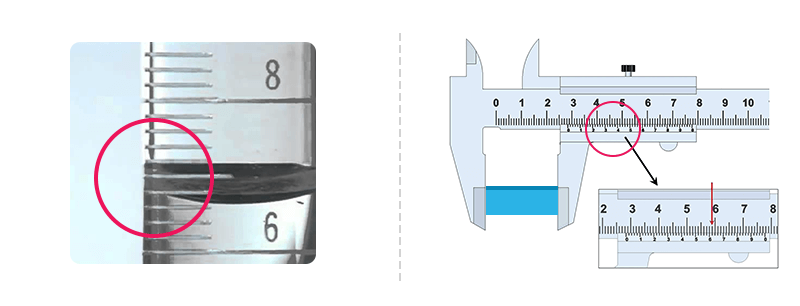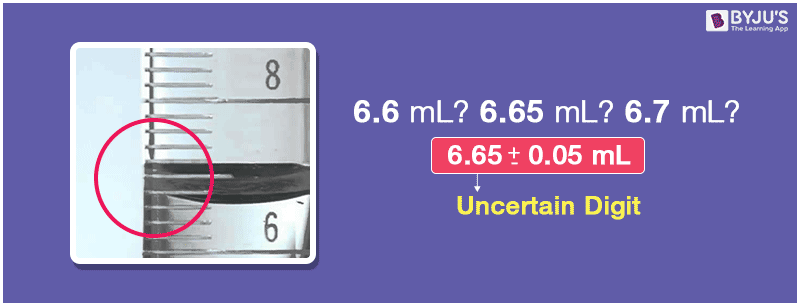# Uncertainty In Measurement

In chemistry, most of the time, we come across both, theoretical as well as experimental calculations. There are many methods which can help in handling these numbers conveniently and with minimal uncertainty.#### Scientific Notation:

Atoms and molecules have extremely low masses, but they are present in large numbers.

Chemists deal with the figures which are as large as $$602,200,000,000,000,000,000,000$$, which is the number of molecules of 2g of hydrogen.

They also have to deal with numbers as small as $$0.00000000000000000000000166g$$, the mass of Hydrogen atom.

There are even other constants like speed of light, charges on particles which have numbers above these stated magnitudes. It is written as ‘SCI’ display mode in scientific calculators.

To help us in handling these numbers we use the following notation: m × 10n, which is, m times ten raised to the power of n. In this n is an exponent having positive and negative values and m is that number which varies from $$1.000…$$ and $$9.999…$$

The scientific notation $$578.677$$ can be written as $$5.78677~ ×~ 10^2$$. In this, the decimal has to be moved left by two places and if it is moved three places left then the power of 10 will be 3.

In the same way $$0.000089$$ can also be written as $$8.9~ ×~ 10^{-5}$$. In this, the decimal is moved five places towards the right, $$(-5)$$ is the exponent in the scientific notation.

This helps us to to attain easier handling, better precision and accuracy while performing operations on numbers with high magnitudes.### Uncertainty in Multiplication and Division:

Same rules can be applied for multiplication and division as well.

For e.g: $$(3.9~ × ~10^6)~×~(2.1~ ×~10^5)$$ = $$(3.9~ ×~ 2.1)(10^{6+5})$$

= $$(3.9 ~×~ 2.1) ~×~ (10^{11})$$

= $$11.31 ~× ~10^{11}$$

$$\frac{3.6~×~10^{-5}}{2.0~×~10^{-4}}$$ = $$(3.6~ ÷~2.0)(10^{-5-(-4)})$$

= $$1.8 ~×~10^{-1}$$

### Uncertainty in Addition and subtraction:

In these operations first of all we have to place these numbers in such a way that they have same exponents.

Therefore, when we add $$5.43 ~×~10^4$$ and $$3.45~×~10^{3}$$, the powers are made equal and after that the coefficients are added and subtracted.

For example: $$5.43 \times 10^4 + 0.345 \times10^5 = (5.43 + (0.345\times 10))\times 10^4$$ = $$8.88~×~10^{4}$$

In the case of subtraction,

$$5.43 \times 10^4 – 0.345 \times10^5 = (5.43 – (0.345\times 10))\times10^4\\$$

$$\\=(5.43 – 3.45)\times10^4 = 1.98 \times 10^4$$